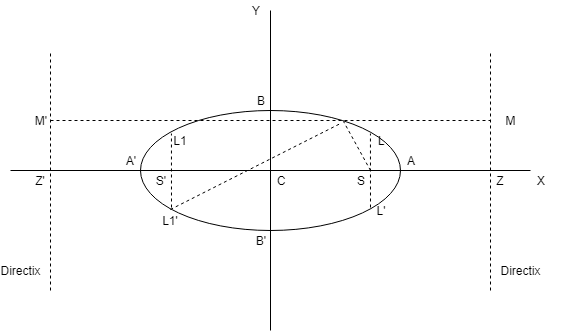# Different Types of Ellipse Equations and Graph

Here, you will learn different types of ellipse and their basic definitions with their graphs.

Let’s begin –

## Different Types of Ellipse

(a)  First type of Ellipse is

$$x^2\over a^2$$ + $$y^2\over b^2$$ = 1, where a > b(a)  AA’ = Major axis = 2a

(b)  BB’ = Minor axis = 2b

(c)  Vertices = ($$\pm a$$, 0)

(d)  Latus rectum LL’ = L1L1′ = $$2a^2\over b$$, equation x = $$\pm$$ae

(e)  Ends of latus rectum are : L(ae, $$b^2\over a$$), L'(ae, -$$b^2\over a$$), L1(-ae, $$b^2\over a$$), L1′(-ae, -$$b^2\over a$$)

(f)  Equation of directrix y = $$\pm a\over e$$

(g)  Eccentricity : e = $$\sqrt{1 – {b^2\over a^2}}$$

(h)  Foci : S = (ae, 0) & S’ = (-ae, 0)

(b)  Second type of Ellipse is

$$x^2\over a^2$$ + $$y^2\over b^2$$ = 1 (a < b)(a)  AA’ = Minor axis = 2a

(b)  BB’ = Major axis = 2b

(c)  Vertices = (0, $$\pm b$$)

(d)  Latus rectum LL’ = L1L1′ = $$2a^2\over b$$, equation y = $$\pm$$be

(e)  Ends of latus rectum are : L($$a^2\over b$$, be), L'(-$$a^2\over b$$, be), L1($$a^2\over b$$, -be), L1′(-$$a^2\over b$$, -be)

(f)  Equation of directrix y = $$\pm b\over e$$

(g)  Eccentricity : e = $$\sqrt{1 – {a^2\over b^2}}$$

(h)  Foci : S = (0, be) & S’ = (0, -be)

#### Line and an ellipse

The line y = mx + c meets the ellipse $${x_1}^2\over a^2$$ + $${y_1}^2\over b^2$$ = 1 in two real, coincident, or imaginary according as $$c^2$$ is < = or > $$a^2m^2 + b^2$$.

Hence y = mx + c is tangent to the ellipse $${x_1}^2\over a^2$$ + $${y_1}^2\over b^2$$ = 1 if $$c^2$$ = $$a^2m^2 + b^2$$

### Related Questions

The foci of an ellipse are $$(\pm 2, 0)$$ and its eccentricity is 1/2, find its equation.

Find the equation of the ellipse whose axes are along the coordinate axes, vertices are $$(0, \pm 10)$$ and eccentricity e = 4/5.

If the foci of a hyperbola are foci of the ellipse $$x^2\over 25$$ + $$y^2\over 9$$ = 1. If the eccentricity of the hyperbola be 2, then its equation is :